Cyber Sale - Only 3 Days Remaining: ALL MCAT Products & Admissions Services

MCAT Content / Circuit Elements / Capacitance

### Capacitance

Topic: Circuit Elements

Capacitance is the measure of an object’s ability to store an electric charge. Any body capable of being charged in any way has a value of capacitance. Capacitors can store energy when a battery or voltage source is connected.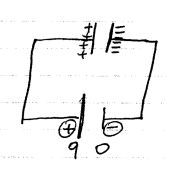A parallel plate capacitor is made up of 2 conducting plates (electrodes), separated by an insulating material (dielectric). When the 2 electrodes are connected to the power supply, one to the positive and one to the negative terminal, there will be a charge build up on the plates. When equilibrium is reached, means that the capacitors are fully charged.For parallel plate capacitors, positive charges accumulated from the connection to the voltage source are attracted to the negative charges accumulated likewise, the charges are stored even when the voltage is removed. Thus energy is stored.

The amount of electric charge stored in each of the plates is directly proportional to the potential difference between the two plates (and of the voltage source):

Q = CV

• Q = Amount of charge stored in one capacitor (+Q on one plate and -Q on the other plate)
• V = Potential difference between the two plates
• C = Capacitance of the capacitor (depends on the shape of the capacitor)

A parallel plate capacitor with a dielectric between its plates has a capacitance is given by the below equation. Note that κ for vacuum is exactly 1. Dielectric constant for air is very close to 1, so that air-filled capacitors act much like those with vacuum.Energy stored in a capacitor is electrical potential energy ΔPE = qΔV. Note that The first charge placed on a capacitor experiences a change in voltage ΔV = 0, since the capacitor has zero voltage when uncharged. The final charge placed on a capacitor experiences ΔV = V, since the capacitor now has its full voltage V on it. The average voltage on the capacitor during the charging process is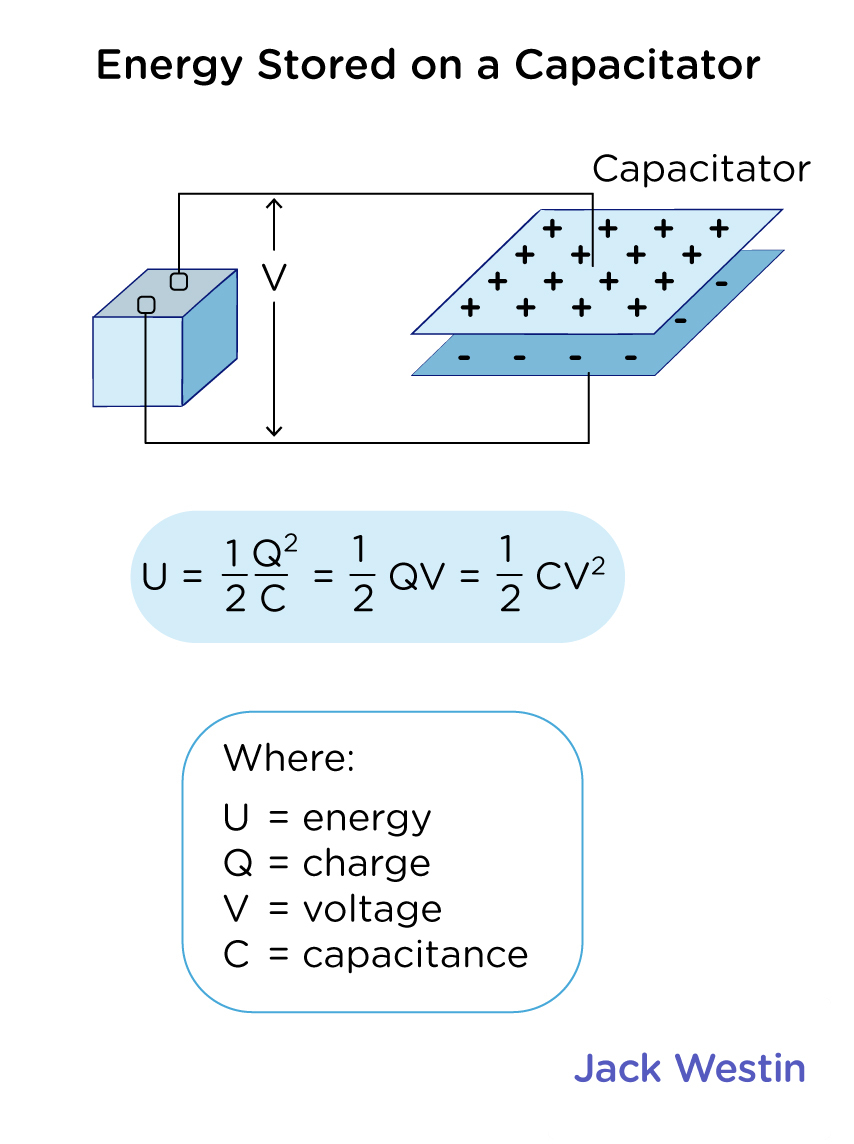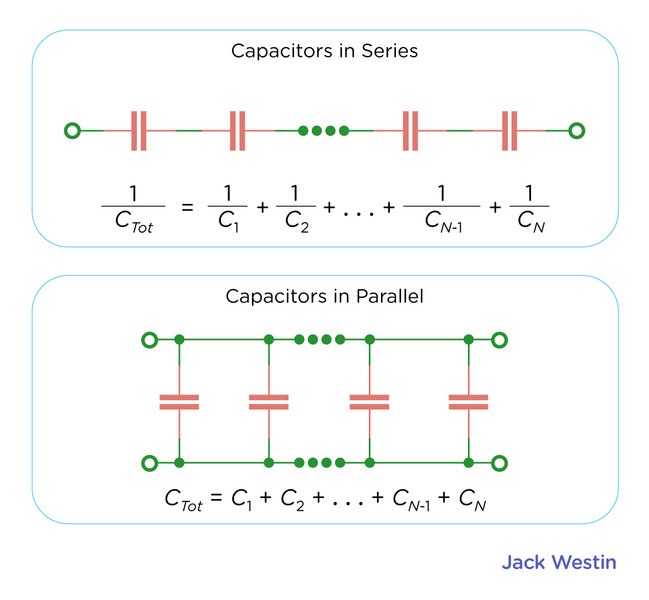Capacitors in series: The total capacitance of in capacitors in series is equal to the sum of the inverse of each individual capacitors.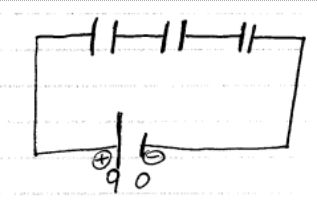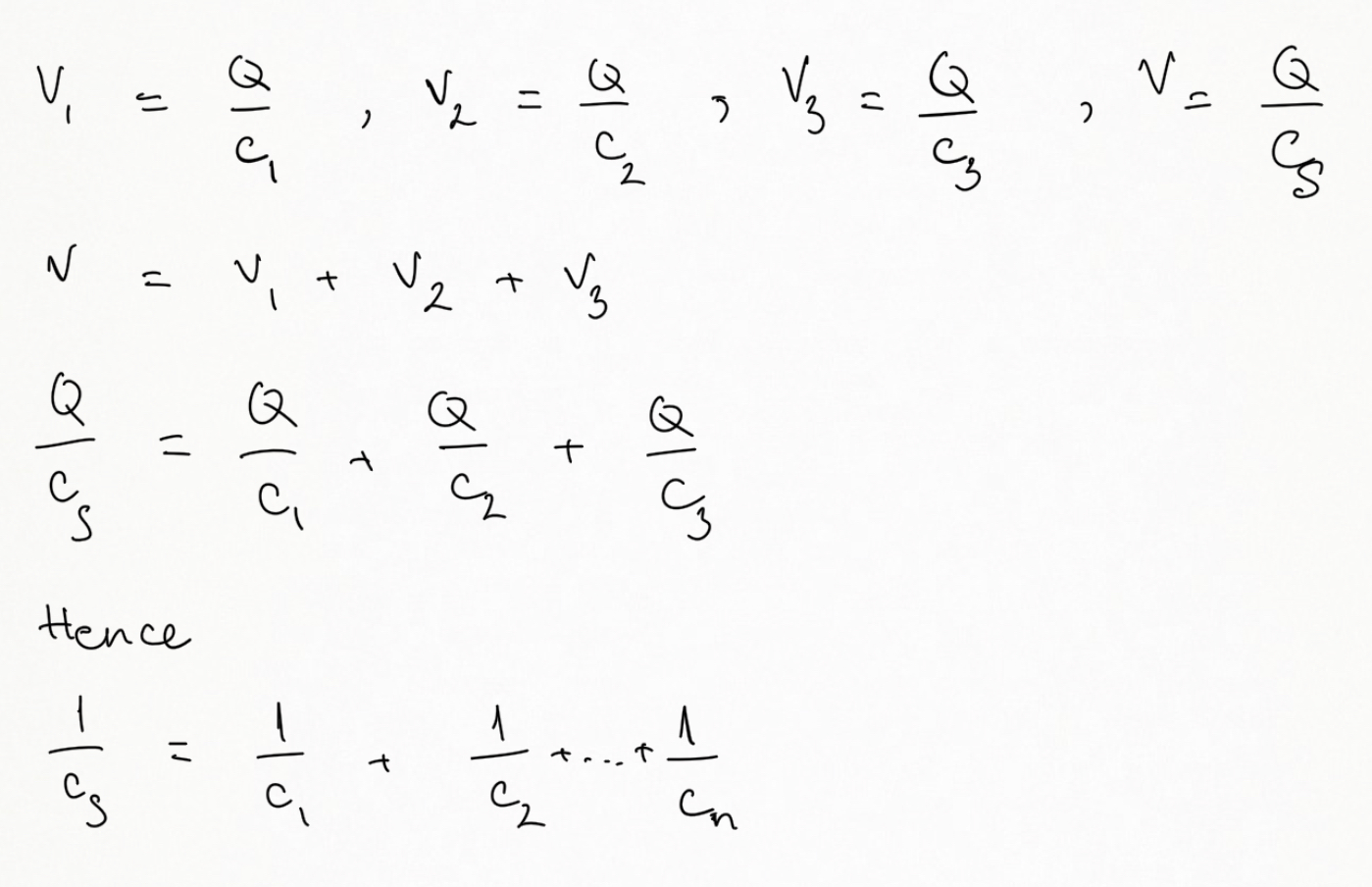Capacitors in parallel: The total capacitance of in capacitors in parallel is equal to the sum of each individual capacitors.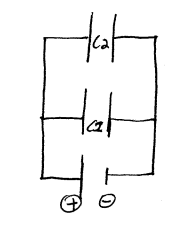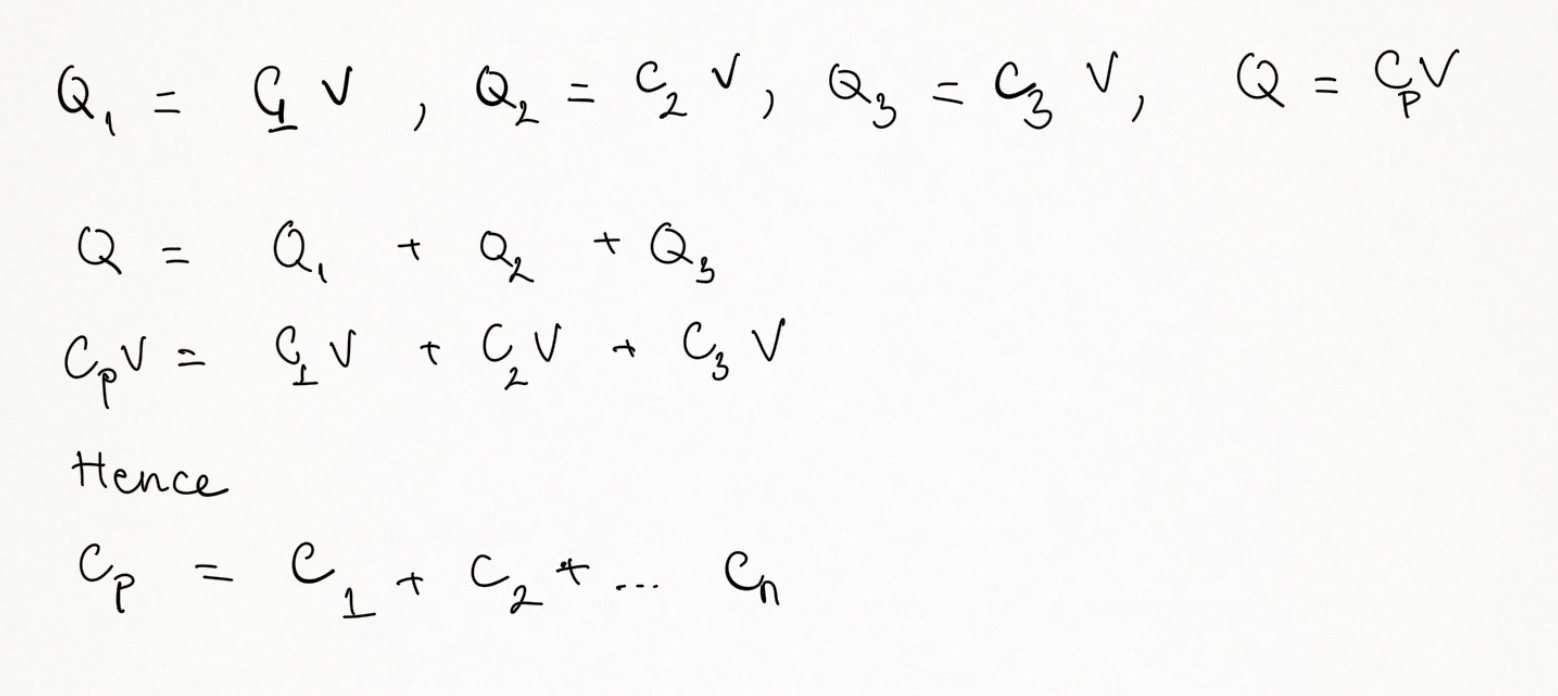In order for a capacitor to hold charge, there must be an interruption of a circuit between its two sides. This interruption can come in the form of a vacuum (the absence of any matter) or a dielectric (an insulator).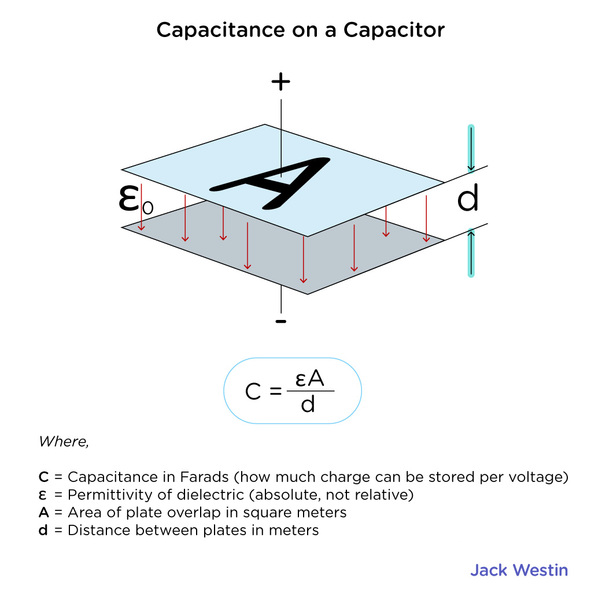When a dielectric is used, the material between the parallel plates of the capacitor will polarize. The part near the positive end of the capacitor will have an excess of negative charge, and the part near the negative end of the capacitor will have an excess of positive charge. This redistribution of charge in the dielectric will thus create an electric field opposing the field created by the capacitor.

Therefore, the net field created by the capacitor will be partially decreased, as will the potential difference across it, by the dielectric. On the other hand, the dielectric prevents the plates of the capacitor from coming into direct contact (which would render the capacitor useless). If it has a high permittivity, it also increases the capacitance for any given voltage.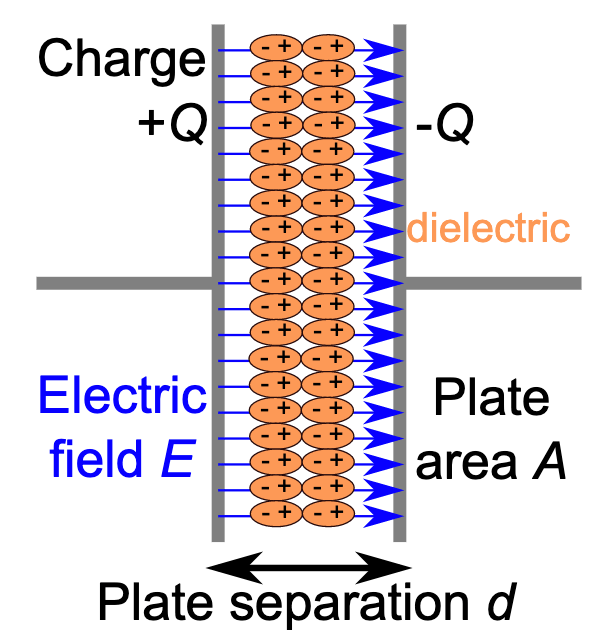Any insulator can be used as a dielectric, but the materials most commonly used are selected for their ability to resist ionization. The more resistant a material is to ionization, the more tolerance it has for operating at higher voltages. Eventually every material has a “dielectric breakdown point,” at which the potential difference becomes too high for it to insulate, and it ionizes and permits the passage of current.

Practice Questions

Capacitors in electrocardiography monitors

Cardiac dysrhythmia and defibrillators

Electric Field Treatments and electroporation

MCAT Official Prep (AAMC)

Physics Question Pack Passage 5 Question 30

Physics Question Pack Passage 5 Question 33

Physics Question Pack Passage 9 Question 53

Physics Question Pack Passage 9 Question 55

Practice Exam 3 C/P Section Passage 2 Question 5

Key Points

• A parallel plate capacitor is made up of 2 conducting plates (electrodes), separated by an insulating material (dielectric).

• A capacitor connected to a voltage source can store energy E = QV/2

• The total capacitance of in capacitors in series is equal to the sum of the inverse of each individual capacitors.

• The total capacitance of in capacitors in parallel is equal to the sum of each individual capacitors.

• Dielectric is the insulating material between the electrodes. With a constant k specific to each type of material.

Key Terms

voltage: Electrical potential difference, expressed in Volts

electrodes: a conductor through which electricity enters or leaves an object, substance, or region.

dielectric: insulating material or a very poor conductor of electric current

electric charge: the physical property of matter that causes it to experience a force when placed in an electromagnetic field

potential difference: the difference in voltage of electrical potential between two points

electric potential energy: is a potential energy that results from conservative Coulomb forces

insulator: A substance that does not transmit heat (thermal insulator), sound (acoustic insulator) or electricity (electrical insulator)

Billing Information